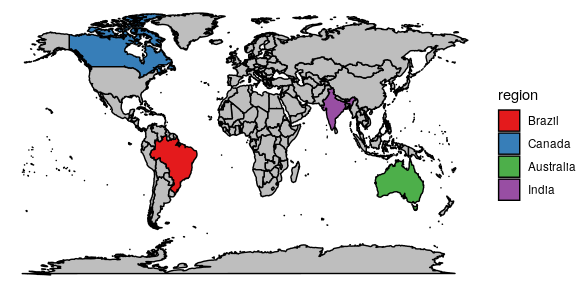# Introduction

ggfocus is a ggplot2 extension that allows the creation of special scales with the purpose of highlighting subgroups of data. The user is able to define what levels of mapped variables should be selected and how the selected subroup should be displayed as well as the unselected subgroup.

### An example

We shall create a sample dataset to be used throughout this guide: the variables u1 and u2 are numeric values and grp is a factor variable with values in A, B, , J.

set.seed(2)
# Create an example dataset
df <- data.frame(u1 = runif(300) + 1*rbinom(300, size = 1, p = 0.01),
u2 = runif(300),
grp = sample(LETTERS[1:10], 300, replace = TRUE))
dplyr::glimpse(df)
#> Observations: 300
#> Variables: 3
#> $u1 <dbl> 0.18488226, 0.70237404, 0.57332633, 0.16805192, 0.94383934, 0.943… #>$ u2  <dbl> 0.37519702, 0.08859551, 0.37619472, 0.22480831, 0.81173888, 0.141…
#> \$ grp <fct> B, B, E, B, J, J, I, A, C, E, C, C, C, A, C, I, B, B, B, H, H, A,…

A natural type of visualization should be mapping u1 and u2 to the x and y axes and mapping grp to color.

ggplot(df, aes(x = u1, y = u2, color = grp)) +
geom_point()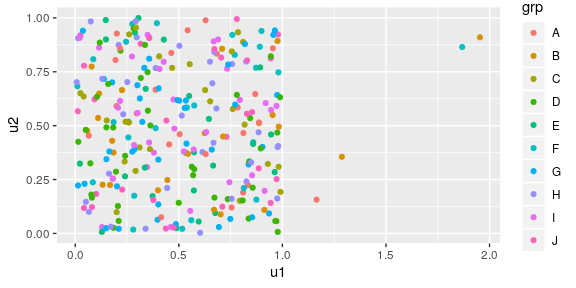### The problem

Suppose you want focus the analysis on the levels A and B. It is not easy to identify where the points are because there is a lot of “noise” in the colors used due to the amount of levels of grp. A simple solution would be filtering out other groups.

library(dplyr)
df %>%
filter(grp %in% c("A", "B")) %>%
ggplot(aes(x = u1, y = u2, color = grp)) +
geom_point()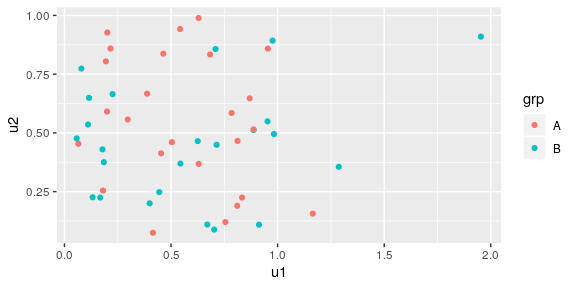While it solves the problems of too many colors making the viewer unable to quickly locate points of A and B and differentiate them, we did lose important information during the filtering, e.g., there are only 4 observations with u1 greater than 1, and 3 of them are in the grp A or B. This is an important information contained in the data that should be considered when the analysis focuses on A and B but require the other observations (a context) in order to be obtained. Therefore, we want to focus on specific levels without taking them out of the context of the data.

### The solution

The solution to focus the analysis in the subgroup and keep the context is to use all the data but group each “unfocused” level in a new level and manipulate scales. This requires data wrangling and scale manipulation.

df %>%
mutate(grp = ifelse(grp %in% c("A", "B"), as.character(grp), "other")) %>%
ggplot(aes(x = u1, y = u2, color = grp)) +
geom_point() +
scale_color_manual(values = c("A" = "red", "B" = "blue", "other" = "gray"))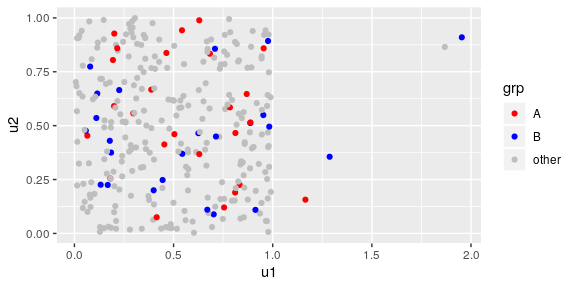This is a solution to the visualization but it required us to:

• use additional data wrangling functions.
• Priorly knowing that the set of colors "red", "blue" and "gray" resulted in a focus on "red" and "blue", therefore the "gray" color is the one that should be used on the unselected group.
• Type more.

### The goal of ggfocus

ggfocus has the goal of creating graphs that focus on a subgroup of the data like the one in the previous example, but without the three drawbacks mentioned. No data wrangling is required (it is all done internally), good scales for focusing on the subgroup are automatically created by default and as a result it is less verbose than selecting scales manually.

Not only color scales are available, but also scales for every other aes in ggplot: fill, alpha, size, linetype, shape, etc. Making it easy to guide the viewer towards the information to focus on using the most appropriate aesthetics for each graph.

The fact that ggfocus manipulates scales only, makes it usable with other extensions of ggplot. Examples using each scale are provided in this guide.

# ggfocus usage

## color and fill

scale_color_focus(focus_levels, color_focus = NULL,
color_other = "gray", palette_focus = "Set1")

scale_fill_focus(focus_levels, color_focus = NULL,
color_other = "gray", palette_focus = "Set1")

color and fill scales have the same default focus scales. They use the color "gray" for unselected observations and the "Set1" palette. Usually, a qualitative color scale is best to visualize the levels focused. The available palettes can be viewed with RColorBrewer::display.brewer.all().

ggplot(df, aes(x = u1, y = u2, color = grp)) +
geom_point() +
scale_color_focus(c("A", "B"))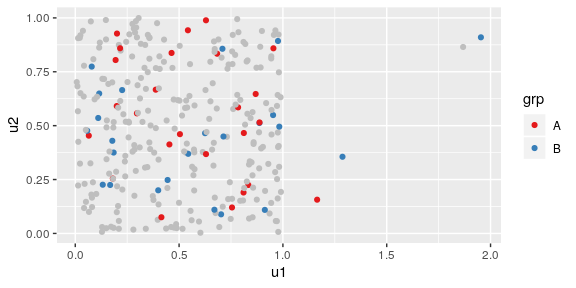ggplot(iris, aes(x = Petal.Length, fill = Species)) +
geom_histogram() +
scale_fill_focus("virginica")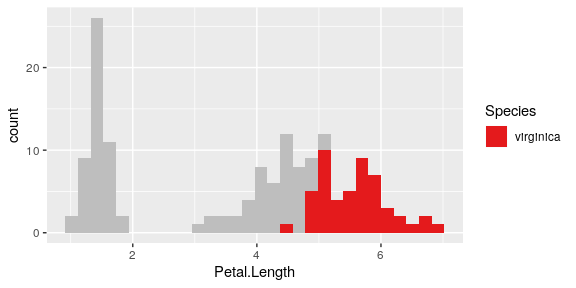One may also use a single color in the color_focus argument to make all the highlighted levels use the same color value. This allows to focus on the subroup as a whole instead of in its individual levels.

ggplot(df, aes(x = u1, y = u2, color = grp)) +
geom_point() +
scale_color_focus(c("A", "B"), color_focus = "red")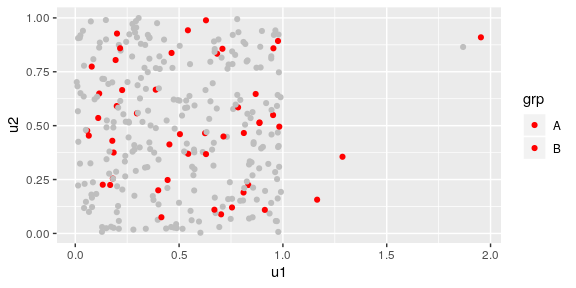## alpha

scale_alpha_focus(focus_levels, alpha_focus = 1, alpha_other = 0.2)

alpha is probably one of the most important aes when drawing focus to specific subroups of your data as the transparency naturally removes the importance given to certain elements. It does not distinguish different groups, therefore it is usually used as a secondary highlighting scale. The argument alpha_other can be used to control the visibility if the unselected observations.

ggplot(df, aes(x = u1, y = u2, alpha = grp)) +
geom_point() +
scale_alpha_focus(c("A", "B")) # Does not distinguish A and B.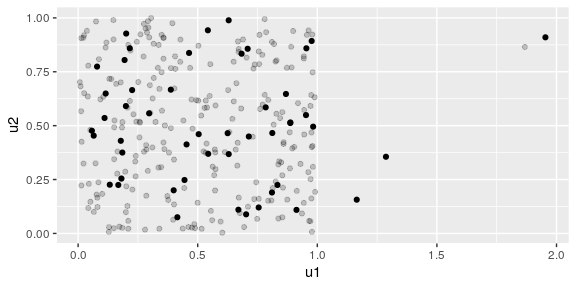ggplot(df, aes(x = u1, y = u2, alpha = grp, color = grp)) +
geom_point() +
scale_alpha_focus(c("A", "B"), alpha_other = 0.5) +
scale_color_focus(c("A", "B")) +
theme_bw() # White background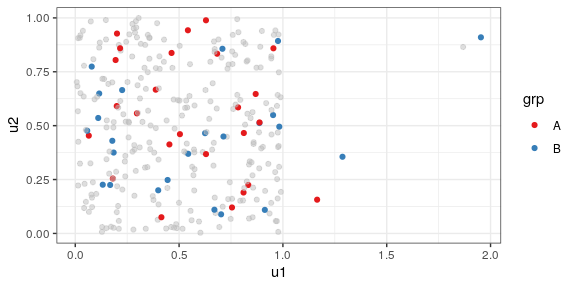## linetype

scale_linetype_focus(focus_levels, linetype_focus = 1, linetype_other = 3)

By default, a continuous line is used for focused levels and dotted line for other levels. Similar to color, one can pass a vector of values in linetype_focus to create different linetypes for each highlighted subgroup although the highest contrast is between continuous and dotted lines.

ggplot(datasets::airquality, aes(x = Day, y = Temp, linetype = factor(Month),
group = factor(Month))) +
geom_line() +
scale_linetype_focus(focus_levels = c(5,7))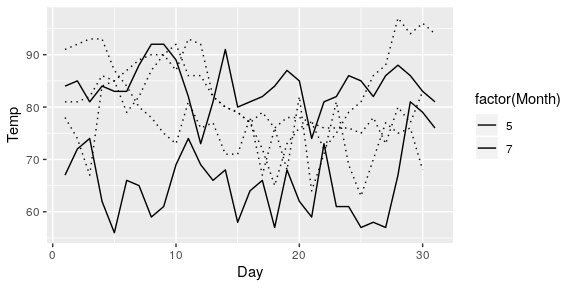ggplot(datasets::airquality, aes(x = Day, y = Temp, linetype = factor(Month),
group = factor(Month))) +
geom_line() +
scale_linetype_focus(focus_levels = c(5,7), linetype_focus = c(1,5))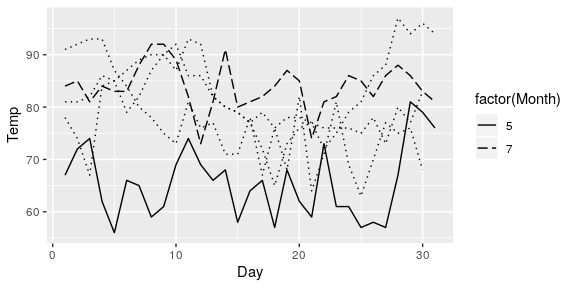## shape

scale_shape_focus(focus_levels, shape_focus = 8, shape_other = 1)

Not to useful to focus on subroups, but it is available. Works just like linetype.

ggplot(df, aes(x = u1, y = u2, shape = grp)) +
geom_point() +
scale_shape_focus(c("A", "B"))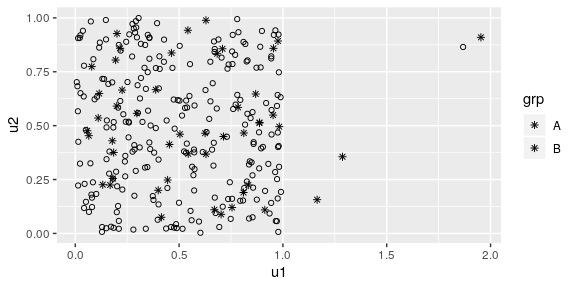ggplot(df, aes(x = u1, y = u2, shape = grp)) +
geom_point() +
scale_shape_focus(c("A", "B"), shape_focus = c(2,3))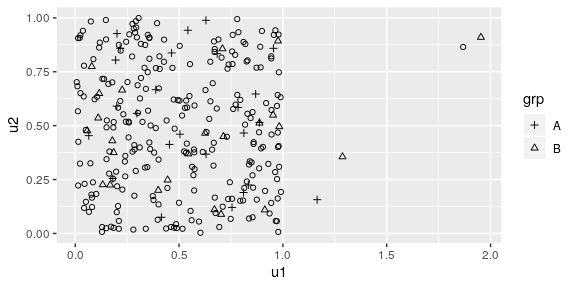## size

scale_size_focus(focus_levels, size_focus = 3, size_other = 1)

Have similar properties as alpha, but using the size of the elements instead to reduce importance instead of transparency.

ggplot(df, aes(x = u1, y = u2, size = grp)) +
geom_text(aes(label = grp)) +
scale_size_focus(c("A", "B"))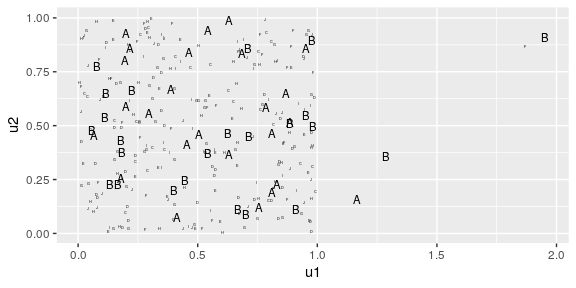ggplot(df, aes(x = u1, y = u2, size = grp, shape = grp)) +
geom_point() +
scale_size_focus(c("A", "B")) +
scale_shape_focus(c("A", "B"))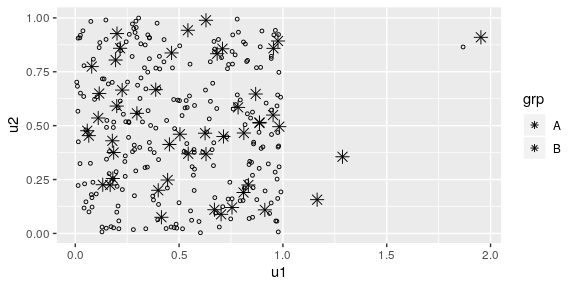## Interaction with other extensions

The main advantage of ggfocus lies in the fact it only manipulates scales to create the focus in the graphs. This fact allows it to interact with other ggplot extensions naturally, as it will work with any type of geom.

Some examples are below:

library(dplyr)
library(ggrepel)
iris %>%
mutate(id = row_number()) %>%
ggplot(aes(x = Petal.Length, y = Sepal.Length, label = id, size = id)) +
geom_text_repel() +
scale_size_focus(c(100,127), size_focus = 8, size_other = 2)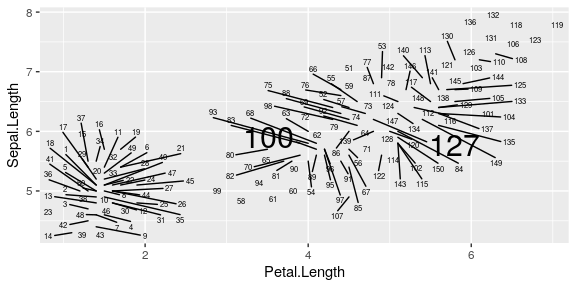library(maps)
wm <- map_data("world")
ggplot(wm, aes(x=long, y = lat, group = group, fill = region)) +
geom_polygon(color="black") +
theme_void() +
scale_fill_focus(c("Brazil", "Canada", "Australia", "India"), color_other = "gray")